# MOSFET â€“ Types and Working Principle

A Metal Oxide Semiconductor Field Effect Transistor (MOSFET) has four terminals − Source (S), Gate (G), Drain (D), Body (B). It is a semiconductor device which is used for switching and amplification applications in electronic circuits. In general, the body terminal is connected with the source thus forming a three terminal device just like an FET.

The MOSFET is a voltage controlled device. Since its operation depends upon the flow of majority carriers only, hence MOSFET is a unipolar device.

In other words, An FET that can be operated in the enhancement mode is known as MOSFET.

Why the Name MOSFET? The gate terminal is insulated from the channel with the help of metal-oxide (SiO2) and hence the name MOSFET

## Types of MOSFET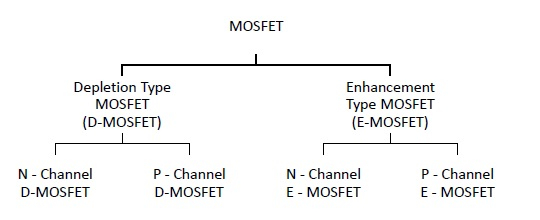## Depletion Type MOSFET

The D-MOSFET can be operated in both depletion mode and enhancement mode. Because of this, it is also known as depletion enhancement MOSFET.

Types of D–MOSFET

D-MOSFETs can be of two types −

• N-channel D-MOSFET
• P-channel D-MOSFET

## N–Channel D–MOSFET

In N–channel D–MOSFET the p–type substrate makes narrower the channel between source and drain so that only small passes remain for the flow of electrons when the drain is made positive with respect to the source.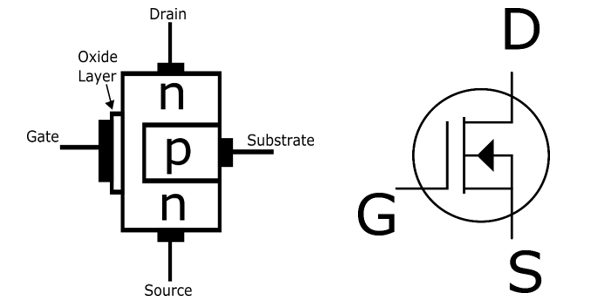### P–Channel D–MOSFET

In this MOSFET, the n–type substrate constricts the channel between the source and drain so that only a small passes remains for the flow of holes from source to drain.## Operation of D–MOSFET Circuit

The gate of D–MOSFET forms a small capacitor. One plate of this capacitor is the gate terminal and the other plate is the channel with the metal oxide layer as the dielectric. When voltage at the gate terminal is changed, the electric field of the capacitor changes that in turn changes the resistance of the conduction channel. Since the gate isolated from the channel by the metal–oxide, hence either positive voltage or negative voltage can be applied to the gate terminal.

When negative voltage is applied to the gate terminal the operation is called depletion mode operation and when positive voltage is applied to gate then operation is called as enhancement mode operation.

### Depletion Mode Operation

Since the gate is at negative potential, hence there are electrons on the gate. These electrons repel the free electrons in the n- channel. So that the n–channel is depleted of some of its free electrons. Thus lesser number of free electrons are available for the current conduction through the channel. This effect is same as the resistance of channel is increased.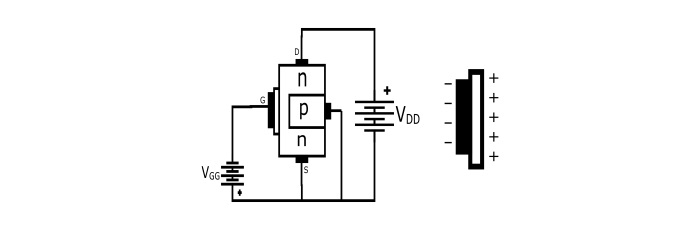The higher the negative voltage at the gate terminal, causes the smaller is the current from source to drain. Hence, by changing the negative voltage on the gate, resistance of the n-channel can be varied and hence the source to drain current.

Since the operation with negative gate depends upon depleting or emptying the channel of free electrons, hence the negative gate operation is known as depletion mode operation.

### Enhancement Mode Operation

Since the gate is positive, it induces negative charges (free electrons) in the channel by the capacitor action. These free electrons are added to those already in the channel, thus the total number of free electrons in the n–channel is increased. Hence a positive gate voltage increases or enhances the conductivity of the channel.

The higher the positive voltage on the gate, grater the current from source to drain. Thus by changing the positive voltage on the gate terminal, the conductivity of channel can be altered.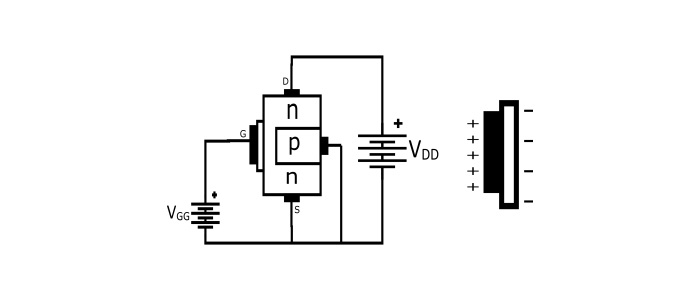Since the operation with positive gate depends upon the enhancing conductivity of the channel, thus the positive gate operation is known as enhancement mode operation.

## Drain Characteristics of D–MOSFET

The drain characteristics of D–MOSFET is the curve between drain current (ID) and Drain–Source Voltage (VDS) for different positive and negative values of gate–source voltage (VGS).

For the positive values of VGS the drain current increases and the D–MOSFET operates in the enhancement mode. Since for n–channel D–MOSFET, the positive gate voltage induces more free electrons in the channel. This increases the drain current and puts the D–MOSFET in the enhancement mode.For the negative value of VGs, the drain current decreases and the D–MOSFET operates in the depletion mode. This is because the negative gate voltage reduces the charge carriers in the n–channel and drain current ID decreases.

It can be seen in the curve, the most of the part of the curve is horizontal, showing constant drain current. Hence in this part the D–MOSFET behaves as a constant current source.

## Enhancement Type MOSFET

The E–MOSFET can be operated in the enhancement mode only. The E–MOSFET has no physical channel from source to drain since the substrate extends completely to the SiO2 layer.

By the application of gate–source voltage (VGS) of proper magnitude and polarity the device can be made operating.

The minimum value of VGS of proper polarity that turns on the E–MOSFET is known as Threshold Voltage (VGS (Th)).

$$\mathrm{For\:the\:n−channel \:E−MOSFET:+V_{GS}\geqslant V_{GS_{th}}}$$

$$\mathrm{For\:the\:p−channel \:E−MOSFETâˆ¶-V_{GS}\geqslant V_{GS_{th}}}$$

## Schematic Symbol of E–MOSFET

### N–Channel E–MOSFET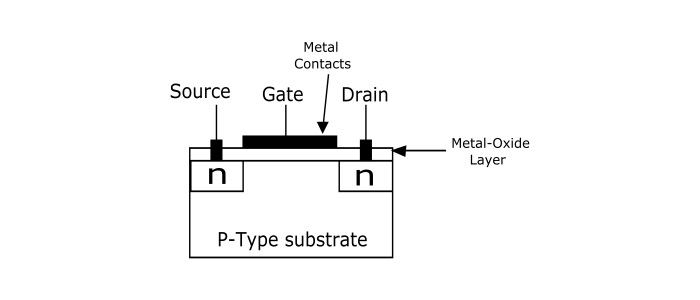### P–Channel E–MOSFET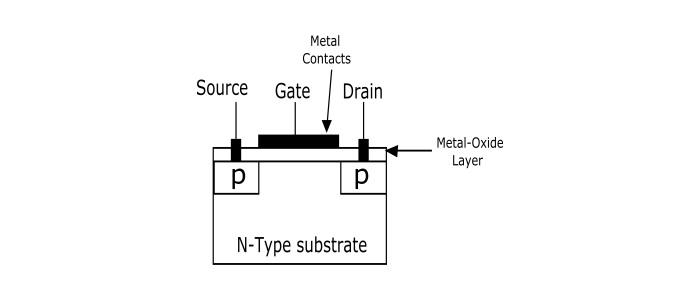### Operation of E–MOSFET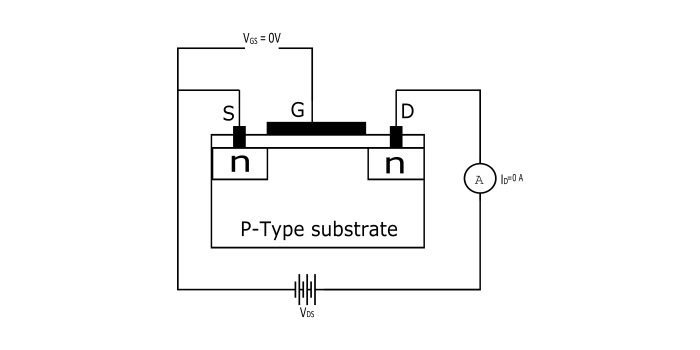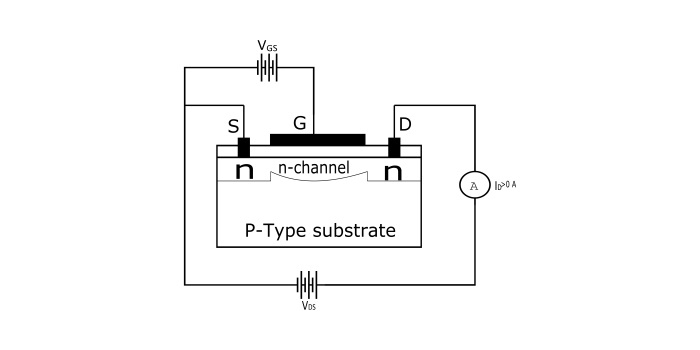When VGS = 0 V, there is no channel connecting the source and drain. The p–type substrate has only a small number of thermally produced free electrons (minority charge carriers) so the drain current is zero. For this reason, The E–MOSFET is generally OFF.

Now when the gate is made positive, it attracts free electrons from p–substrate. The free electrons combine with the holes just below the SiO2 layer. If VGS is positive enough, all the holes touching the SiO2 layer are filled and remaining free electrons start flowing from source to drain. This is same as creating a thin layer of n–type material below the SiO2 layer. Hence the E–MOSFET is turned ON and drain current starts flowing from source to drain.

## Drain Characteristics of E–MOSFET

The drain characteristics is the graph between drain current and drain–source voltages for the various positive values of VGS. From the graph, it can be seen that most of the part of the curve is horizontal, showing constant drain current. Hence in this part of curve the E–MOSFET behaves as a constant current source.## Applications of MOSFET

• MOSFET Amplifiers are widely used in radio frequency applications.

• Speed of DC Motors can be regulated with help of Power MOSFET.

• Due to high switching speed, it can be used in chopper circuits.